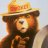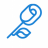# RMI scanner

T

#### tutianlong

##### New member
VIP
I have done up a RMI indicator for my chart so it will give a signal when RMI is above overbought point or oversold point.

Code:
``````input length = 20;
input momentum = 3;
input Overbought_Point = 70;
input Oversold_Point = 25;

Assert(momentum > 0, "'momentum' must be positive: " + momentum);

def na = Double.NaN;
def emaInc = ExpAverage(Max(close - close[momentum], 0), length);
def emaDec = ExpAverage(Max(close[momentum] - close, 0), length);

def RMI = 100 - 100 / (1 + emaInc / emaDec);

declare lower;

#Supertrend arrow signal
plot SuperTrendUP = if rmi < oversold_point then 1 else 0;
plot SuperTrendDN = if rmi > overbought_point then 1 else 0;``````
How can i tweak the code for it t scan stocks whose RMI values go above the designated overbought or oversold points?How do I convert this ATR% chart study into a scanner watchlist column? Questions 4Scanner is using older candles than what is intended Questions 0